# OECD Non-Financial Corporations Debt to Surplus Ratio Analysis 3 - Calculating Confidence Interval in R, Parametric and Monte Carlo.UnsplashHeather Wildeが撮影した写真

This post is following of the above post. In this post, I will show some statistics of our data.

Before investigation, I make data frame to wide format with pivot_wider() function.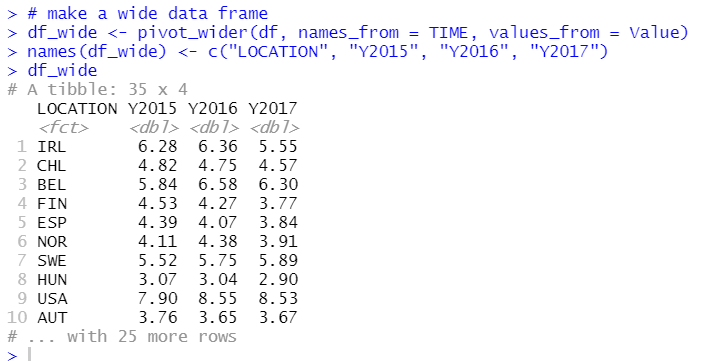With above data frame, df_wide, let's check some basic statistics for each year.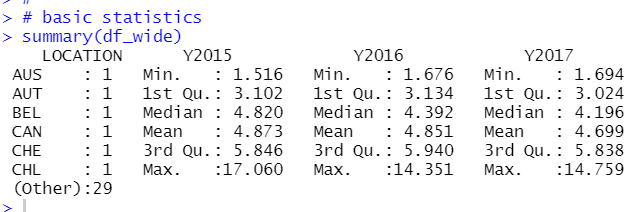The avarage value for 2015 is 4.873, the average value for 2016 and the average value for 2017 is 4.699. So, there is a slight down trend.

Let's calculate confindence interval of those averages.

To calculate Confidence Interval, I need number of observations, average and standard deviation.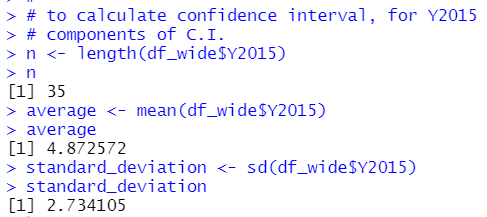Then, I can calculate C.I.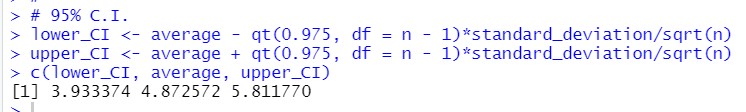So, for 2015, debt to surplus ratio is average 4.873, 95% confidence interval is from 3.933 to 5.811.

Let's calclulate C.I. for 2016 and 2017. Before calculating, I make a custom function to calculate C.I.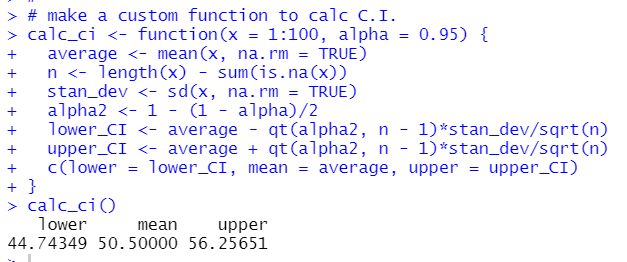Then, using this custom function, let's caclulate Confidence Interval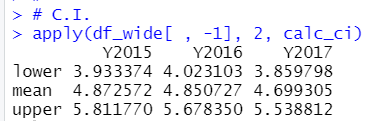You can confirm that 2015 figure are same as previous calculation.

Above confidence interval comes from parametric method because I use parameters such as number of observations, average and standard deviation.

Next, let's get confidence interval with Monte Carlo simulation.

I repeat 10,000 to calculate average and get 95% interval.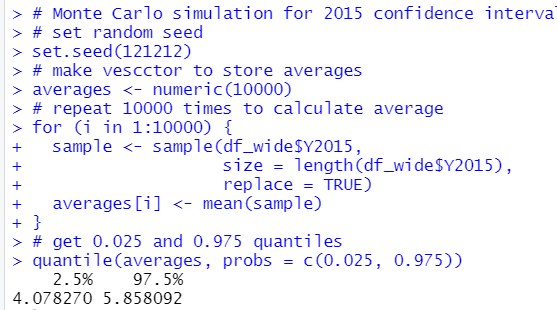For 2015, Monte Carlo simulation 95% confidence interval is from 4.078 to 5.858. This is very close to parametric confidence interva, 3.933 to 5.11.

Let's calculate confidence interval for 2016 and 2017 too.

Before calculation, I make a custom function to do Monte Carlo simulation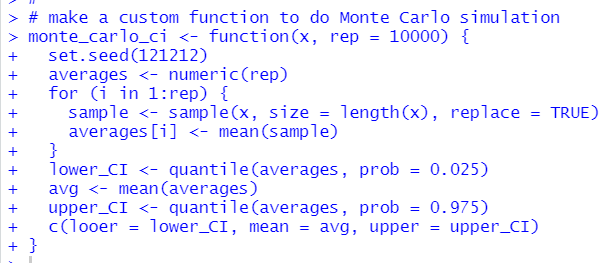Then, I calculate confidence intervals with Monte Carlo simulation.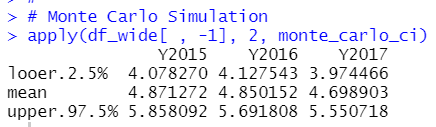You can confirm 2015 confidence interval is the same as previous calculation.

Let's compare parametric C.I. and Monte Carlo C.I.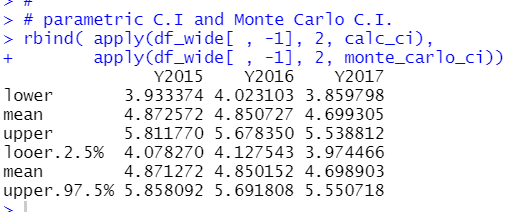First 3 rows are parametric C.I. and Bottom 3 rows are Monte Carlo C.I.

You will find Monte Carlo C.I. are shiftted to greater values.
The reason is Debt to Surplus Ration has skewed to right.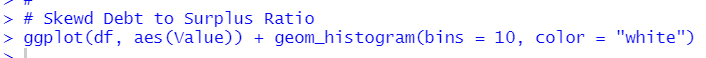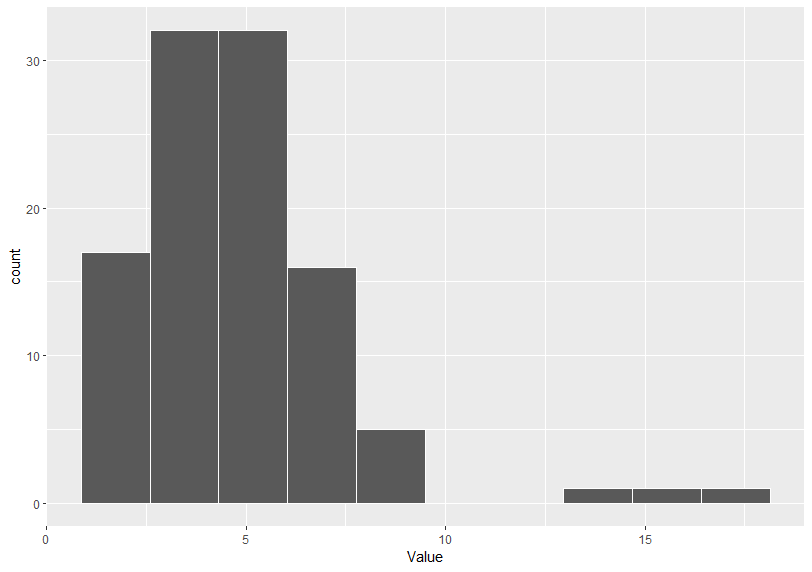That's it. Thank you!

The next post is